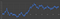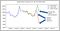# The Stratified Cox Proportional Hazards Regression Model

## A tutorial on how to build a stratified Cox model using Python and Lifelines

The Cox proportional hazards model is used to study the effect of various parameters on the instantaneous hazard experienced by individuals or ‘things’.

1. All individuals or things in the data set experience the same baseline hazard rate.

After training the model on the data set, you must test and verify these assumptions using the trained model before accepting the model’s result.

# Schoenfeld Residuals: The idea that turned regression modeling on its head

## What are they? How to use them to test the assumptions of the Cox Proportional Hazards model?

One thinks of regression modeling as a process by which you estimate the effect of regression variables X on the dependent variable y. Your model is also capable of giving you an estimate for y given X. You subtract that estimate from the observed y to get the residual error of regression.

But what if you turn that concept on its head by estimating X for a given y and subtracting that estimate from the observed X?

That’s right —you estimate the regression matrix X for a given response vector y!

When you do such a thing, what you get are the Schoenfeld Residuals named after their inventor David Schoenfeld who in 1982 showed (to great success) how to use them to test the assumptions of the Cox Proportional Hazards model. …

# A two-sentence description of Survival Analysis

Survival Analysis lets you calculate the probability of failure by death, disease, breakdown or some other event of interest at, by, or after a certain time. While analyzing survival (or failure), one uses specialized regression models to calculate the contributions of various factors that influence the length of time before a failure occurs.

# What is it used for?

In medicine, survival analysis is used to measure the efficacy of drug and vaccine candidates in randomized controlled trials. …

# The Fascinating Math Powering the COVID-19 Vaccine Trials

## An overview of techniques and math used in vaccine studies

With the COVID-19 pandemic raging, big pharma, small pharma, medium sized pharma — pharmaceutical companies of any size with an idea for a vaccine and the funding to pursue it — are racing to get the vaccine out to the physician’s desk, and to get the world out of its nightmare.

It’s against this backdrop, that the world got a rare look at the intricate workings of the massive COVID-19 vaccine trials being conducted by Moderna, Pfizer and AstraZeneca. …

# Regression with ARIMA Errors

## What is it, why do we need it, when to use it, how to build it using Python and statsmodels

Regression with ARIMA errors combines two powerful statistical models namely, Linear Regression, and ARIMA (or Seasonal ARIMA), into a single super-powerful regression model for forecasting time series data.

The following schematic illustrates how Linear Regression, ARIMA and Seasonal ARIMA models are combined to produce the Regression with ARIMA errors model:Surely this isn’t just a random process! Or is it? (Image by Author)

# The White Noise Model

## The most important statistical model

White noise are variations in your data that cannot be explained by any regression model.

And yet, there happens to be a statistical model for white noise. It goes like this for time series data:

# Assumptions of Linear Regression

## And how to test them using Python.

Linear Regression is the bicycle of regression models. It’s simple yet incredibly useful. It can be used in a variety of domains. It has a nice closed formed solution, which makes model training a super-fast non-iterative process.

A Linear Regression model’s performance characteristics are well understood and backed by decades of rigorous research. The model’s predictions are easy to understand, easy to explain and easy to defend.

If there only one regression model that you have time to learn inside-out, it should be the Linear Regression model.

If your data satisfies the assumptions that the Linear Regression model, specifically the Ordinary Least Squares Regression (OLSR) model makes, in most cases you need look no further. …Sales forecast generated using Holt-Winters Exponential Smoothing (Data source: US FRED) (Image by Author)

# Holt-Winters Exponential Smoothing

## A super-fast forecasting tool for time series data

Holt-Winters Exponential Smoothing is used for forecasting time series data that exhibits both a trend and a seasonal variation. The Holt-Winters technique is made up of the following four forecasting techniques stacked one over the other:

# What is time series decomposition and how does it work?

## Plus a headfirst dive into a powerful time series decomposition algorithm using Python

A time series can be thought of as being made up of 4 components:

A seasonal component
A trend component
A cyclical component, and
A noise component.

## The Seasonal component

The seasonal component explains the periodic ups and downs one sees in many data sets such as the one shown below.

# When Your Regression Model’s Errors Contain Two Peaks

## A Python tutorial on dealing with bimodal residuals

A raw residual is the difference between the actual value and the value predicted by a trained regression model.